﻿ Application of Complex Rotation Method for Calculate to Correlation Factor and the 2sns<SUP>+1,3</SUP>S<SUP>e</SUP>, 2snp<SUP>+1,3</SUP>P<SUP>0</SUP>, 2pnp<SUP>+1,3</SUP>D<SUP>e</SUP>, 3d4d <SUP>+1,3</SUP>G<SUP>e</SUP> Autoionizing States of He-like AtomsPublications are Open
Access in this journal
Article Versions
Export Article
• Normal Style
• MLA Style
• APA Style
• Chicago Style
Research Article
Open Access Peer-reviewed

### Application of Complex Rotation Method for Calculate to Correlation Factor and the 2sns+1,3Se, 2snp+1,3P0, 2pnp+1,3De, 3d4d +1,3Ge Autoionizing States of He-like Atoms

B. Sow , A. Diouf, Y. Gning, B. Diop, M. Sow, A. Wague
International Journal of Physics. 2018, 6(6), 174-180. DOI: 10.12691/ijp-6-6-1
Received October 14, 2018; Revised November 24, 2018; Accepted December 09, 2018

### Abstract

Calculations of the energy levels of atoms and ions with Z ≤ 10 are carried out in this paper using the complex rotation method. Using a new wave function with two terms including a new method to calculate the correlation factor taking into account spherical harmonics through hypergeometric functions to calculate excited 2sns+1,3Se, 2snp+1,3P0, 2pnp+1,3De, 3d4d +1,3Ge states. The results obtained show quantitatively the great significance of electron correlation effects in the doubly excited states. These results are in compliance with some experimental and theoretical data.

### 1. Introduction

The study of atomic resonances in plasma environment has gained tremendous attention in the recent years and is one of the interesting topics of current research 1, 2, 3, 4. The complex rotation method was used in atomic physics by Doolen 5 to determine the resonance parameters in the system e- + H and by Lonnie W. Manning and Frank C. Sanders 6 to study the resonance parameters (1P0 and 3P0) of He-like systems. Subsequently other calculations were made; using the variational wave functions built on a Hylleraas basis, by Ho 7, 8, 9, 10, Gning et al 11 used the Hylleraas wave functions to compute resonance parameters. Some of his work 7 focused on the determination of the energies and widths of the first two resonances 1Se and 1,3P0 of helium and the H- and Li+ ions under the excitation threshold n = 4. Similarly the complex rotation method allows calculating positions and widths of resonances in atomic systems 12, 13, 14. To mention just a few applications, the method has been used in nonrelativistic quantum-mechanical calculations of resonance parameters of doubly excited states 15, 16 or Stark resonances 17 in such systems as He, H-, or Ps-. The above mentioned applications of the complex rotation procedure neglected relativistic effects. Recent developments of the experimental technique perfected experimental data to a degree where relativistic effects become observable.

A number of methods based on close-coupling approximation 18 and the Feshbach projection operator formalism 19, 20 have been used to calculate positions and widths of doubly excited states. In the latter method, resonance positions and widths are calculated separately and therefore need not be of the same accuracy.

In this work we report a resonance parameters calculation for some lower-lying doubly excited states for a He-like systems below the n = 2 hydrogenic thresholds. Hylleraas-type wave functions are used and 2sns+1,3Se, 2snp+1,3P0, 2pnp+1,3De, 3d4d +1,3Ge states are calculated.

### 2. Wave Functions and Calculations

The existence of this term makes impossible to solve exact the fundamental equation of quantum mechanics (Schrödinger equation):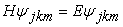(1)

In this equation,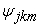is the wave function of the quantum state of the system (core-electrons) and E is the total energy of the same quantum state. The exact solution of the last equation is impossible because of the correlation term involved in the expression of total Hamiltonian. Hence it is necessary to implement a method of approximate calculation using a correlated wave function, which is given by: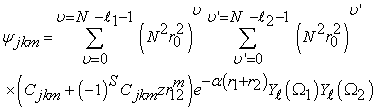(2)

Where j+k+m ≤ Ω, Ω is a positive integer number which determines the expansion length N; α is a nonlinear parameter and S the total spin of atomic system, r1 and r2 are the coordinates of electrons with respect to the nucleus; N is the principal number ; ℓ1 and ℓ2 are orbital angular, Z the nuclear charge. Cjkm are parameters to be determined, r0 is Bohr radius.

Where(3)

represent the spherical harmonics of electron 1 or 2.

Which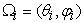(4)

and i= 1, 2.

The resonance parameters are determined by finding a rate of change that is stable with respect to the nonlinear parameters α, and the complex value of the energy.(5)

where Er gives the resonance position andgives the width.

And the Hamiltonian can be written as:(6)

With(7)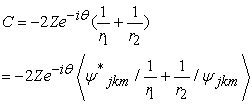(8)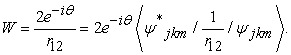(9)

In equation (6), T is the kinetic energy; C is the Coulomb interaction between the atomic nucleus and the two electrons and W is the Coulomb interaction between electrons. In the study of atomic resonances, the application of this theorem consists in the transformation of real Hamiltonian system H(r) to a complex one H(r,θ) where r is the inter-particle radial coordinate and θ is a positive real number denoting the rotation angle. This processing is executed by a change of the real variable r to complex variable r exp (iθ).

The matrix element of the normalization constantis defined by: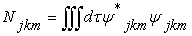(10)

Whereis elementary volume element. The existence of the disturbance term (Equation 9) makes impossible the exact resolution of equation (1). This is how we used the complex rotation method by introducing a new method of calculating the correlation coefficient, which is one of the calculation techniques for providing the resonance parameters to two-electron atomic systems. According to the calculation of correlation coefficients, proposed by Varshalovich D.A et al. 32, we found a new formula that is proposed by Gning et al. 11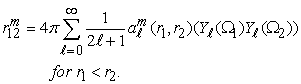(11)

With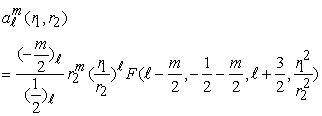(12)

the hypergeometric wave function defined by Samarskogo 33(13)

Replacing eqaution (13) with its value in equation (12)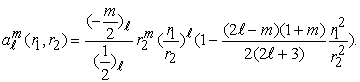(14)

So equation (14) becomes(15)

And remplacing equation (15) in equation (11)(16)

for 2sns+1,3Se states (ℓ=0;S=0),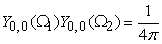(17)

so equation (2) becomes:(18)

For 2snp+1,3P0, 2pnp+1,3De states corresponding to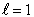,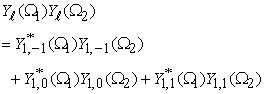(19)

by replacing the values of the spherical harmonics of e- 1or 2, we obtain:(20)

For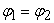we have=1(21)

For 3d4d +1,3Ge states(22)

### 3. Results and Discussions

In our calculations, we set the dimension Ω to 4 for the 2s3s 1,3Se, 2s4s 1,3Se states and β=0.25 rad. Ω =3 for the 2s2p 1,3P0, 2s3p 1,3P0 states and β=0.2 rad. Similarly β=0.2 rad for the 2p3p 1,3De, 2p4p 1,3De, 3d4d 1,3Ge states and Ω =4. After having fixed these parameters, we have varied the nonlinear parameter α. The minimum value of the energy is obtained following a stabilization of the latter with a variation of the nonlinear parameter. At each minimum value of the energy corresponds a minimum value of the given resonance width 10-3. Based on the processing of the calculations, energy conversion of 1 a.u. = 2Ry = 27.211385 eV was used.

Table 1, Table 2, Table 3, Table 4 show the results of targeted energy 2s3s 1,3Se, 2s4s 1,3Se, 2s2p 1,3P0, 2s3p 1,3P0, 2p3p 1,3De, 2p4p 1,3De, 3d4d 1,3Ge respectively.

Based on the processing of the calculations, energy conversion of 1 a.u. = 2Ry = 27.211385 eV was used. For the calculation of doubly excited states for Helium-like ions, several authors have used different methods. Thus, Ho 21, 29 used the method of complex rotation convoluted with a wave function of Hylleraas type, Dieng 22 used Special Forms of Hylleraas-Type Wave Functions, Ivanov and Safronova 24 performed calculation using double sum method over the complete hydrogen spectrum, Sakho 23, 25 with the Screening Constant by Unit Nuclear Charge method, Ivanov and Safronova 24 using computing double sum method, Bachau 30 using the Fechbach projection formalism method.

In fact, a comparison is made in Table 5 based on the theoretical results from Ho 21, Dieng 22, Sakho et al. 23, 25 and Ivanov and Safronova 24 for the 2s3s 1,3Se, 2s4s 1,3Se states. For these state, best consistency of the results was found and a little discrepancies regard Dieng results.

Table 6 compares results with those from Ho 8, 21, Seminario and Sanders 26, Drake et al 27, Sakho et al. 23, 28, Ivanov and Ho 29, Bachau 30 for the 2s2p 1,3P0 , 2s3p 1,3P0 For these states, we note generally a quite good agreement.

In Table 7, results of 2p3p1,3De, 2p4p1,3De states were compared with those of Ho 21, Sakho et al. 25 and Dieng 22. For these states, we note generally a quite good agreement and little discrepancy with Dieng results.

We have also compared in Table 8, results with those from Ivanov and Ho 29 and Bachau 30 for the 3d4d1Ge, 3d4d3Ge. We note good consistency.

In Table 8 our resonance width results of the same states above are listed, correspondingly, and also compared with those found in the literature Ho 8, 21, Seminario and Sanders 26, Manning and Sanders 31.

### 4. Conclusion

We have carried out calculations of the inter-shell nℓnand nℓn(with n 3, n4 and = or) singlet and triplet doubly excited states energies of helium-like ions by using the complex rotation method and new wave functions with two terms are used to calculate resonance parameters for intrashell states. The results of the resonance parameters reported here agree better with other theoretical calculations and experiment data for low Z than those with high Z. The results that we have obtained show that it is possible by using our theoretical approach, to describe the highly doubly excited two-electrons states. However, experimental techniques using synchrotron radiation are becoming more sophisticated and can provide very accurate values of the resonance parameters of the Rydberg series of multi-electron atomic systems. We can always use the method of complex rotation and with weak wave functions of terms to validate these experimental results. So this work also provides parameters for total widths for such resonances. Since the widths are related to the inverse of the autoionisation Jifetimes, the present results should be useful for future investigations of the underlying mechanism of autoionisation.

### References

  Y. K. Ho and S. Kar, Few-Body Syst. 53, 437. (2012). In article View Article  L. G. Jiao and Y. K. Ho, Phys. Plasmas 20, 083303. (2013). In article View Article  A. F. Ordonez-Lasso, J. C. Cardona, and J. L. Sanz-Vicario, Phys. Rev. A 88, 012702. (2013). In article View Article  P. Jiang, S. Kar, and Y. Zhou, Phys. Plasmas 20, 012126. (2013). In article View Article  Doolen G. D., A procedure for calculating resonance eigenvalues; J. Phys. B: Atom. Molec. Phys. 8, 525. (1975). In article View Article  Lonnie W. Manning and Frank C. Sanders; Complex rotation method applied to Z-dependent perturbation theory: The 2s2p autoionizing states of two-electron atoms; Phys. Rev. A 44, 11. (1991). In article  Ho Y. K. and Callaway J.,Doubly excited of helium atoms between the N=2 and N=3 He+ thresholds.J. Phys. B,.18: p. 3481. (1985). In article View Article  Ho Y. K. and Bathia A. K., Complex-coordinate calculation of 1,3D resonances in two electrons systems.Phys. Rev. A,. 44: p. 2895. (1991). In article View Article  PubMed  Ho Y. K., Strong electric-field effects on the doubly-excited intrashell states of He below the He+ (N=2) thresholds., Z. Phys. D, 38: p. 191-196. (1996). In article View Article  Ho Y. K., Doubly-excited 1,3P0autoionization states of He between the N=4 and N=6 thresholds of He+ ions. Z. Phys. D, 42: p. 77-81. (1997). In article View Article  Youssou Gning, et al. Calculations of resonances parameters for the ((2s2) 1Se, (2s2p) 1,3P0) and ((3s2) 1Se, (3s3p) 1,3P0) Doubly excited states of helium-like ions with z≤10 using a complex rotation method implemented in Scilab. Radiation Physics and Chemistry 106 ,1-6. (2015) In article View Article  Ho Y.K. Phys. Rep. 99, 1 (1983). In article View Article  A. Maquet, Shih-I. Chu, and W.P. Reinhardt, Phys. Rev. A 27, 2946. (1983). In article View Article  W.P. Reinhardt, Annu. Rev. Phys. Chem. 33, 223. (1982). In article View Article  Ho Y.K. Phys. Rev. A 44, 4154. (1991). In article View Article  PubMed  I.A. Ivanov and Y.K. Ho, Phys. Rev. A 61, 032501. (2000). In article View Article  Ho Y.K. and I.A. Ivanov, Phys. Rev. A 63, 062503. (2001). In article View Article  Oza D H Phys. Rev. A 33 824. (1986). In article View Article  Bhatia A K and Temkin A. Phys. Rev. A 36 1050. (1964). In article  Bhatia A K and Temkin A. Phys. Rev. A 29 1895. (1984). In article View Article  Ho Y. K., Complex-coordinate calculations for doubly excited states of two-electron atoms .Phys. Rev. A,.23: p. 2137. (1981). In article  Dieng M , Biaye M., Gning Y., The Inter-Shell Doubly Excited 1,3Se, 1,3Po, 1,3De, 1,3Fo, and 1,3Ge States Energies Calculations of Helium-Like Ions Using Special Forms of Hylleraas-Type Wave Functions Chin. J. Phys. 51, 4. (2013). In article View Article  Sakho, I, Konte, A., Ndao, A. S, Biaye, M. and Wague, A. Investigation of 2snp±1,3Po resonances oftwo-electron systems using the screeningconstant by unit nuclear charge method phys. Scr. 77. (2008). In article  Ivanov I. A. and SafronovaU.I .Opt. Spectrosc.75 506 (1993). In article  Sakho, I. Screening constant by unit nuclear charge calculations of 1,3Se, 1,3Po and 1,3De intershell Rydberg states of the helium-like ions below the N = 2 hydrogenic thresholds, Eur. Phys. J. D 61, 267-283 (2011). In article View Article  Seminario J. M., Sanders F. C., Application of Z-dependent perturbation theory to autoionizing states of helium like atoms: Feshbach projection method; Phys Rev A 42, 2562. (1990). In article View Article  PubMed  Drake G. W. F. and Dalgarno A., A 1/Z expansion study of the 2s2p 1P and 3P autoionizing resonances of the helium isoelectric sequence; Proc. Roy. Soc. Lond. Scr A 320 549 (1971). In article View Article  Sakho, I. Screening constant by unit nuclear charge calculations for (ns2) 1Se, (np2) 1De and (Nsnp) 1P0 excited states of He-like systems., Eur. Phys. J. D 47, 37-44, (2008). In article View Article  Ivanov I. A. and Ho Y. K., Hylleraas Basis Calculation of the Doubly Excited 1,3Ge States in Helium like Ions, Chin. J. Phys. 39, 415. (2001). In article  Bachau, H., Martin, F., Riere, A., Yanez, M. At. Data Nucl. Data Tables 48, 167. (1991). In article View Article  Manning, L.W., Sanders, F.C. Phys. Rev. A 44, 11. (1991). In article View Article  Varshalovich, D.A., et al., Quantum Theory of Angular Momentum. Scientific Publishing Co. Ltd. (1988). In article View Article  Samarskogo, A. A Base de la Théorie des Fonctions Spéciales. Edition Science, Moscow, 281 p. (1974). In article

Published with license by Science and Education Publishing, Copyright © 2018 B. Sow, A. Diouf, Y. Gning, B. Diop, M. Sow and A. WagueThis work is licensed under a Creative Commons Attribution 4.0 International License. To view a copy of this license, visit http://creativecommons.org/licenses/by/4.0/